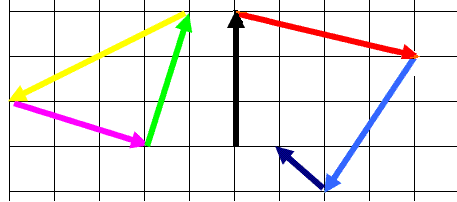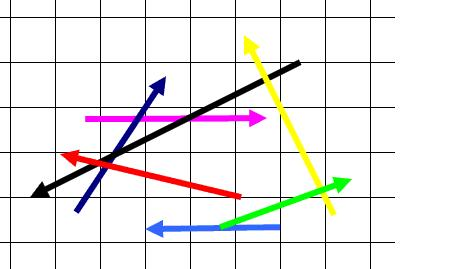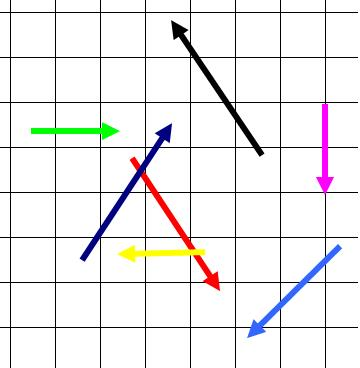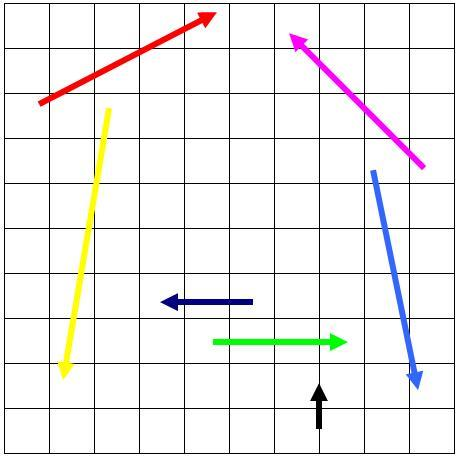#### You may also like### Building Tetrahedra

Can you make a tetrahedron whose faces all have the same perimeter?A 1 metre cube has one face on the ground and one face against a wall. A 4 metre ladder leans against the wall and just touches the cube. How high is the top of the ladder above the ground?Four rods are hinged at their ends to form a convex quadrilateral. Investigate the different shapes that the quadrilateral can take. Be patient this problem may be slow to load.

# Spotting the Loophole

##### Age 14 to 16Challenge Level

In 2D, vectors may be thought of as arrows with a fixed length and direction. The place at which the arrow starts is not important, so they may be translated around the plane without affecting the value of the vector.

This allows us to add and subtract vectors visually: if the vectors can be translated so as to form a closed circuit then the vector sum is zero. If the vectors cannot be translated so as to form a closed circuit then their vector sum is not zero, as in this diagram in which the vectors on the left have zero sum, whereas the vectors on the right don't have zero sum.In this problem we are given three grids of vectors. The vectors are only represented visually, but it is assumed that their x and y values are whole numbers (Remember: the x and y values represent the horizontal and vertical offsets from the start of the arrow, not the location of the start of the arrow itself).

Your task, in each case is to try to see which subsets of the vectors form closed loops.

In each grid can you find a closed loop of vectors?

In each case is the closed loop unique? You can prove your assertions using algebra or a convincing visual argument.

Try hard to solve using visualisation before resorting to algebra.

Grid 1:Grid 2Grid 3